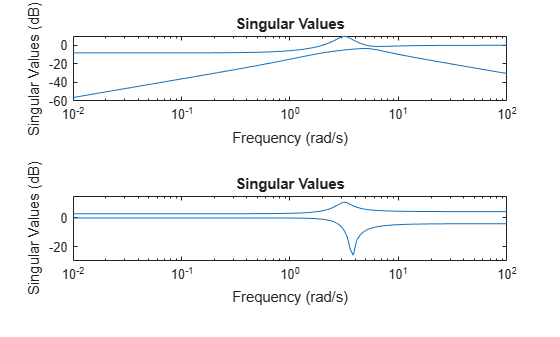# sigma

Singular values plot of dynamic system

## Syntax

```sigma(sys) sigma(sys,w) sigma(sys,[],type) sigma(sys,w,type) sigma(sys1,sys2,...,sysN,w,type) sigma(sys1,'PlotStyle1',...,sysN,'PlotStyleN',w,type) sv = sigma(sys,w) [sv,w] = sigma(sys) ```

## Description

`sigma ` calculates the singular values of the frequency response of a dynamic system `sys`. For an FRD model, `sigma` computes the singular values of `sys.Response` at the frequencies, `sys.frequency`. For continuous-time TF, SS, or ZPK models with transfer function H(s), `sigma` computes the singular values of H(jω) as a function of the frequency ω. For discrete-time TF, SS, or ZPK models with transfer function H(z) and sample time Ts, `sigma` computes the singular values of

`$H\left({e}^{j\omega {T}_{s}}\right)$`

for frequencies ω between 0 and the Nyquist frequency ωN = π/Ts.

The singular values of the frequency response extend the Bode magnitude response for MIMO systems and are useful in robustness analysis. The singular value response of a SISO system is identical to its Bode magnitude response. When invoked without output arguments, `sigma` produces a singular value plot on the screen.

`sigma(sys)` plots the singular values of the frequency response of a model `sys`. This model can be continuous or discrete, and SISO or MIMO. The frequency points are chosen automatically based on the system poles and zeros, or from `sys.frequency` if `sys` is an FRD.

`sigma(sys,w)` explicitly specifies the frequency range or frequency points to be used for the plot. To focus on a particular frequency interval `[wmin,wmax]`, set `w = {wmin,wmax}`. To use particular frequency points, set `w` to the corresponding vector of frequencies. Use `logspace` to generate logarithmically spaced frequency vectors. Frequencies must be in `rad/TimeUnit`, where `TimeUnit` is the time units of the input dynamic system, specified in the `TimeUnit` property of `sys`.

`sigma(sys,[],type)` or `sigma(sys,w,type)` plots the following modified singular value responses:

 `type = 1` Singular values of the frequency response H–1, where H is the frequency response of `sys`. `type = 2` Singular values of the frequency response I + H. `type = 3` Singular values of the frequency response I + H–1.

These options are available only for square systems, that is, with the same number of inputs and outputs.

`sigma(sys1,sys2,...,sysN,w,type)` plots the singular value plots of several LTI models on a single figure. The arguments `w` and `type` are optional. The models `sys1,sys2,...,sysN `need not have the same number of inputs and outputs. Each model can be either continuous- or discrete-time.

`sigma(sys1,'PlotStyle1',...,sysN,'PlotStyleN',w,type)` specifies a distinctive color, linestyle, and/or marker for each system plot. See `bode` for an example.

`sv = sigma(sys,w)` and ```[sv,w] = sigma(sys)``` return the singular values `sv` of the frequency response at the frequencies `w`. For a system with `Nu` input and `Ny` outputs, the array `sv` has `min(Nu,Ny)` rows and as many columns as frequency points (length of `w`). The singular values at the frequency `w(k)` are given by `sv(:,k)`.

## Examples

collapse all

Consider the following two-input, two-output dynamic system.

`$H\left(s\right)=\left[\begin{array}{cc}0& \frac{3s}{{s}^{2}+s+10}\\ \frac{s+1}{s+5}& \frac{2}{s+6}\end{array}\right].$`

Compute the singular value responses of H(s) and I + H(s).

```H = [0, tf([3 0],[1 1 10]) ; tf([1 1],[1 5]), tf(2,[1 6])]; [svH,wH] = sigma(H); [scIH,wIH] = sigma(H,[],2);```

In the last command, the input 2 selects the second response type, I + H(s). The vectors `svH` and `svIH` contain the singular value response data, at the frequencies in `wH` and `wIH`.

Plot the singular value responses of both systems.

```subplot(211) sigma(H) subplot(212) sigma(H,[],2)```## Tips

You can change the properties of your plot, for example the units. For information on the ways to change properties of your plots, see Ways to Customize Plots.

## Algorithms

`sigma` uses the MATLAB® function `svd` to compute the singular values of a complex matrix.

For TF, ZPK, and SS models, `sigma` computes the frequency response using the `freqresp` algorithms. As a result, small discrepancies may exist between the `sigma` responses for equivalent TF, ZPK, and SS representations of a given model.